# Economics: Overview

This is an Insight article, written by a selected partner as part of GCR's co-published content. Read more on Insight

## In summary

In cases alleging that prices have been inflated by anticompetitive conduct, overcharges are often assessed by comparing prices during the alleged conduct period to prices before or afterward, while controlling for other factors that influence price using a multivariate regression model. If a control variable that trends over time is measured imperfectly, however (as is often the case for cost), then the multivariate regression method may systematically misestimate overcharges. Although this phenomenon results from well-established econometric principles, its practical significance makes it important for practitioners to understand. We walk through a stylised example in which prices are determined competitively, yet an overcharge regression finds a positive, statistically significant overcharge. Note that it is also possible for measurement error to mask the extent of an overcharge when an overcharge does exist. We then explain the statistics underlying this phenomenon, provide intuition about the direction of the bias on the overcharge estimate, and discuss potential solutions.

## Discussion points

• Measurement error in a control variable can cause bias in overcharge estimates
• A number of methods are available to detect and potentially mitigate measurement-error bias

• Overcharge regression and estimation
• Measurement error and measurement-error bias
• Attenuation bias

## Measurement-error bias in overcharge calculations

In cases alleging that anticompetitive conduct has inflated prices, the amount of price inflation (the overcharge) is commonly estimated using multivariate regression. Overcharge regressions are used to justify claims for billions of dollars in damages, so it is particularly important that they produce reliable estimates. Yet a common data limitation can cause multivariate regression to misestimate overcharges – and even to find large and statistically significant overcharges where none actually exist.

The chief attraction of multivariate regression is its potential ability to separate conduct-driven price inflation from other influences on prices. To do so, a typical overcharge regression seeks to measure the effect of the alleged conduct while controlling for other factors (called control or explanatory variables) that explain variations in price. But perfect data on these factors is rarely available, so experts hired to estimate overcharges routinely rely on imperfect data.

If producers of the product at issue adjust their prices in response to changes in their production costs, for instance, then it may be important for the overcharge regression to control for the relevant production costs. In the likely event that perfect data on each firm’s production costs is not available, the expert may turn to whatever cost data is available – for example, to a cost index that abstracts from firm- and product-specific variation in production cost.1 In effect, this available but imperfect cost data is a mismeasured version of the ‘true’ cost that determines these producers’ prices. Although such mismeasurement may appear small, random and benign, it can actually predispose the overcharge regression to meaningfully misstate overcharges.2

In this article, we demonstrate how measurement error in an explanatory variable that is trending over time, such as cost, can cause a regression approach to misestimate overcharges. For simplicity, we lead with an example in which the true overcharge is zero by construction, but measurement error nonetheless leads to the appearance of a positive overcharge – a ‘phantom overcharge’. Conversely, it is also possible for measurement error in an explanatory variable to mask the extent of an overcharge when an overcharge does exist.

The observation that measurement error can bias overcharge estimates does not break new econometric ground. Econometricians are well aware that mismeasurement of an explanatory variable can bias estimates in a regression model, and that the direction of the bias may be upwards or downwards, depending on the circumstances.3 But it is important that practitioners also understand that overcharge estimates can be biased by measurement error, as these estimates can have powerful real-world consequences. To this end, we present guidance on when measurement error is likely to result in upward- or downward-biased overcharge estimates and briefly review some possible methods for mitigating this bias.

## A stylised example

We begin by illustrating the phantom-overcharge phenomenon using a stylised example. The result we illustrate here could be generated by measurement error in any control variable, but for ease of explanation, we focus on cost. Cost is a good candidate both because it is an important determinant of price and because available cost data is typically imperfect (ie, measured with error). We refer to the correct cost that influences price as the ‘true cost’ and the imperfect cost data available to the expert as ‘measured cost’.

In our stylised example, we simulate quarterly data for the years 2011–2014. We assume that prices are determined competitively, with the competitive price in quarter t given as:

priceₜ= 10 + true costₜ 

That is, the price in each quarter is exactly equal to the true cost plus \$10. Figure 1 shows that costs and prices move in lockstep over time, each increasing by \$1 every quarter.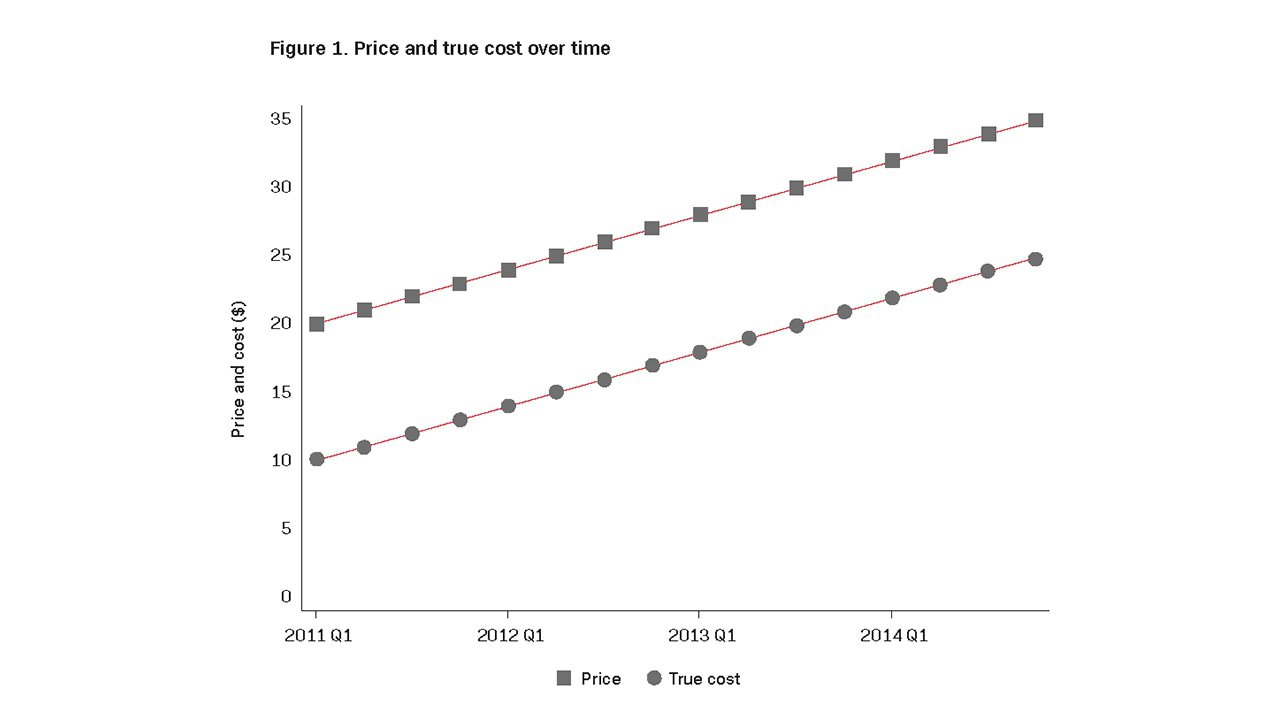Now imagine allegations that a conspiracy began artificially inflating prices in 2013. An expert is hired to estimate overcharges. The expert has access to data on price and cost, but the cost data is imperfect: in any given quarter, the measured cost may be higher or lower than true cost by as much as \$3.50, which is 20 per cent of the mean true cost across the years 2011–2014.4 Although cost is measured with error, note that the measured cost does not systematically overstate or understate true cost. The data available to the expert is shown in Figure 2.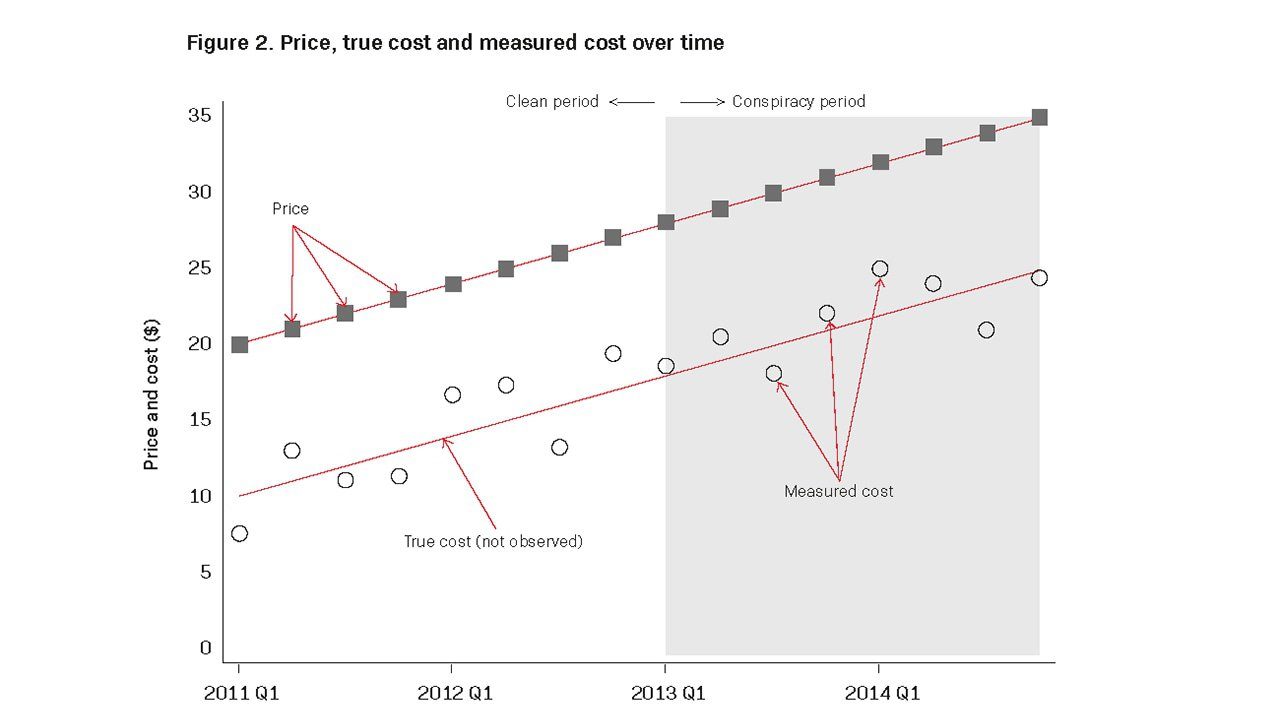The availability of data before and during the alleged conspiracy lends itself to two common approaches for estimating overcharges: the prediction approach and the dummy-variable approach. As we show below, both approaches are vulnerable to the appearance of phantom overcharges.

### The prediction approach

In the prediction approach,5 the expert starts by estimating the relationship between price and cost during the ‘clean’ period prior to the alleged conspiracy. To do so, he runs a regression of price on measured cost (the hollow circles in Figure 2) using data only from the ‘clean’ years, 2011 and 2012, and finds:

ˆpriceₜ = 16.50 + (0.52 x measured costₜ) 

Note that the expert estimates that in the ‘clean’, non-conspiratorial world, price tends to increase by 52 cents when measured cost rises by \$1. This estimated relationship is weaker than the 1:1 relationship that actually exists between price and true cost. As we explain below, the weakness of this estimated relationship results from measurement error and is key to the appearance of phantom overcharges (or, under other circumstances, to the underestimation of overcharges).

The next step in the prediction approach is to use the estimated ‘clean’ relationship between price and cost to predict what prices would have been in 2013 and 2014 had there not been a conspiracy. The expert applies the estimated relationship from equation  to the measured cost data for 2013 and 2014. He or she then calculates the difference between the actual 2013 and 2014 prices and his or her prediction of what those prices would have been but for the alleged conspiracy:

Table 1. Overcharge calculation using the prediction approach

QuarterActual Price (\$)But-For Price (\$)Implied Overcharge (\$)Implied Overcharge (%)
2013 Q128.0026.061.946.9
2013 Q229.0026.982.026.9
2013 Q330.0025.744.2614.2
2013 Q431.0027.803.2010.3
2014 Q132.0029.302.708.4
2014 Q233.0028.744.2612.9
2014 Q334.0027.206.8020.0
2014 Q435.0029.006.0017.1
Average31.5027.603.9012.4

As shown in Table 1, the prediction approach suggests that there are positive overcharges in all quarters of the alleged conspiracy period. Even though prices are determined competitively throughout this period and the true overcharge is zero by construction, the mismeasurement of cost will lead the expert to report that a standard econometric approach has found overcharges averaging 12.4 per cent. For every billion dollars in sales, this implies phantom overcharges totalling approximately \$124 million.6

### The dummy-variable approach

In the dummy-variable approach, the expert sets up a multivariate regression model to examine the following question: after controlling for cost, how much higher were prices during the alleged conspiracy period than during the clean period? This regression model has two explanatory variables: (1) measured cost and (2) a dummy variable equal to 0 during the clean period (2011 and 2012) and equal to 1 during the alleged conspiracy period (2013 and 2014). Using all four years of data to estimate this model, the expert finds:

ˆpriceₜ = 15.89+ (0.56 x measured costₜ) + (3.54 x conspiracy dummyₜ) 

In other words, the expert estimates that price increases 56 cents for every \$1 increase in cost; and that after controlling for cost, prices tended to be \$3.54 higher during the alleged conspiracy period than during the clean period. The estimated coefficient on the conspiracy-period dummy, \$3.54 (or, on average, 11.2 per cent of price during the conspiracy period), is the expert’s estimate of the overcharge, and it is statistically significant. Even though the true overcharge is zero, the expert will report that a standard econometric approach has identified statistically and economically significant overcharges. In fact, for every billion dollars in sales, this approach yields phantom overcharges totalling approximately \$112 million.

While these results may seem surprising – or, perhaps, a chance occurrence particular to this stylised example – they are, in fact, neither. Rather, they are a predictable and generalisable outcome of relying on an imperfect cost measure to control for the effect of true cost on price in a scenario such as this.

## The statistics underlying measurement-error bias

The key to understanding how these two standard regression methods lead to the appearance of phantom overcharges lies in the coefficient on cost. Notice that in the examples above, the estimated coefficients on cost (0.52 and 0.56) are less than one, even though there is a perfect 1:1 relationship between price and true cost. This disparity arises because of the measurement error in the available cost data, which muffles the apparent relationship between price and cost.

This muffling is a well-known phenomenon in econometrics and statistics. In technical parlance, the phenomenon is called ‘attenuation bias’, meaning that the measurement error in the cost data puts a downward bias on the estimated magnitude of the effect of cost on price.7

For an intuitive understanding of the phenomenon, look back at the example above, in which price and true cost move in lockstep: for every \$1 increase in the true cost, the price increases by \$1 as well. Because of measurement error, however, the price does not move in lockstep with measured cost. As the price and true cost increase by \$1, for example, the measured cost might increase by only 50 cents, or it might increase by \$1.50. Whether the measured cost increases by 50 cents or \$1.50, however, the price continues to rise one-for-one with the \$1 increase in true cost. In other words, a portion of the variation in measured cost does not represent variation in true cost, and therefore does not provoke any variation in price. As a result, we underestimate the 1:1 relationship between price and cost.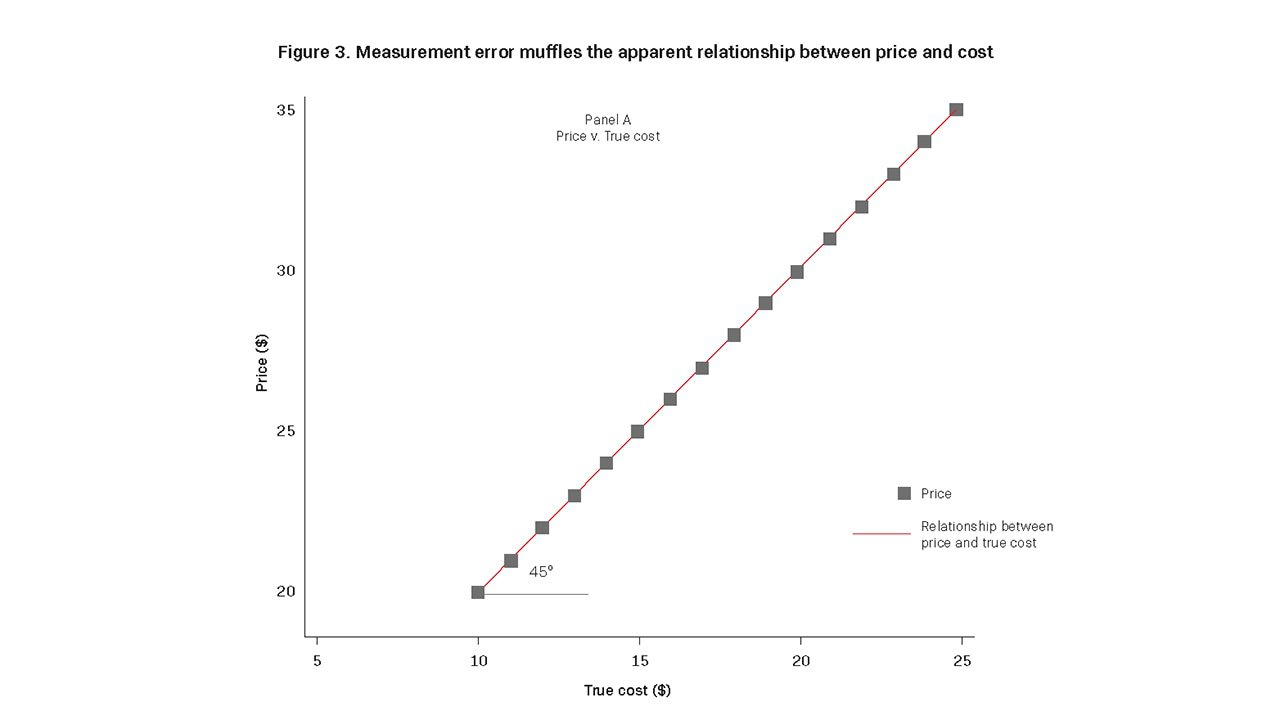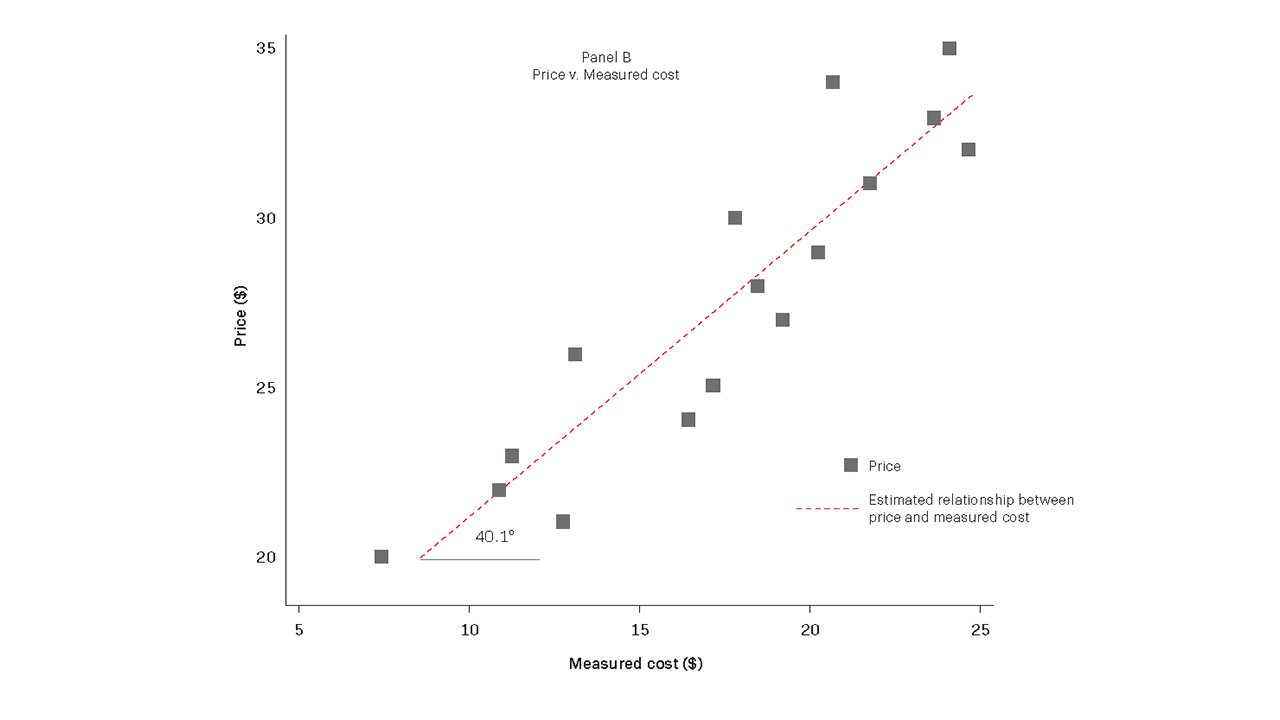Figure 3 illustrates this underestimation. In Panel A, price is plotted against true cost, and the line fitted to these points reflects the true 1:1 relationship between price and cost. In Panel B, price is plotted against measured cost, and the best-fit line to these points has a slope shallower than the 45 degrees corresponding to a 1:1 relationship.

Underestimating the relationship between price and cost has a knock-on effect on overcharge estimates. To see this, remember that in our stylised example, true cost is rising over time. In the context of the prediction approach, the expert’s underestimation of the price-cost relationship therefore means that he or she fails to fully account for the effect of rising costs on prices.

This failure is illustrated in Figures 4A and 4B. Figure 4A shows actual prices and the clean-period prices predicted by the expert’s regression. Although the predicted and actual prices are on average about the same during the clean period, the expert’s underestimation of the price-cost relationship during that period leads him or her to underpredict the upward trend in prices driven by the upward trend in costs. As a result, the trend in predicted prices (indicated by the dashed line) has a shallower slope than do actual prices. As Figure 4B shows, this shallower slope translates into an underprediction of prices during the conspiracy period. Although the rise in true cost causes prices to increase from an average of \$23.50 during the clean period to an average of \$31.50 during the conspiracy period – a difference of \$8.00 – the expert uses the underestimate of the price-cost relationship to predict that the rising cost should cause a price increase of only \$4.10. The expert then ascribes the difference between actual prices and the underpredicted prices to the alleged conspiracy. This difference is the phantom overcharge.

A similar failure occurs in the context of the dummy-variable approach. Here, again, the expert underestimates the effect of cost on price, and therefore finds that cost does not fully explain the increase in price from the clean period to the alleged conspiracy period. The conspiracy-period dummy variable is left to ‘explain’ the remaining price increase, and thus picks up a positive coefficient. Although the conspiracy-period dummy variable is merely picking up the slack for the mismeasured cost variable, the expert incorrectly concludes that its positive coefficient is proof of antitrust damages.

Generally, a bias on the estimated effect of one explanatory variable in a multivariate regression – such as bias arising from measurement error – implies a bias on the estimated effects of all explanatory variables.8 These biases can become complex if further explanatory variables are added to the model or if the expert switches to a more sophisticated estimation method. In the simple case where price is regressed on cost and a conspiracy-period dummy, however, there will be a positive bias on the estimated effect of the conspiracy-period dummy (ie, a bias towards finding a positive overcharge or overstating an existing overcharge) when measured cost and the conspiracy-period dummy are positively correlated.9, 10 Conversely, when measured cost and the conspiracy-period dummy are negatively correlated, there will be a bias towards understating the overcharge.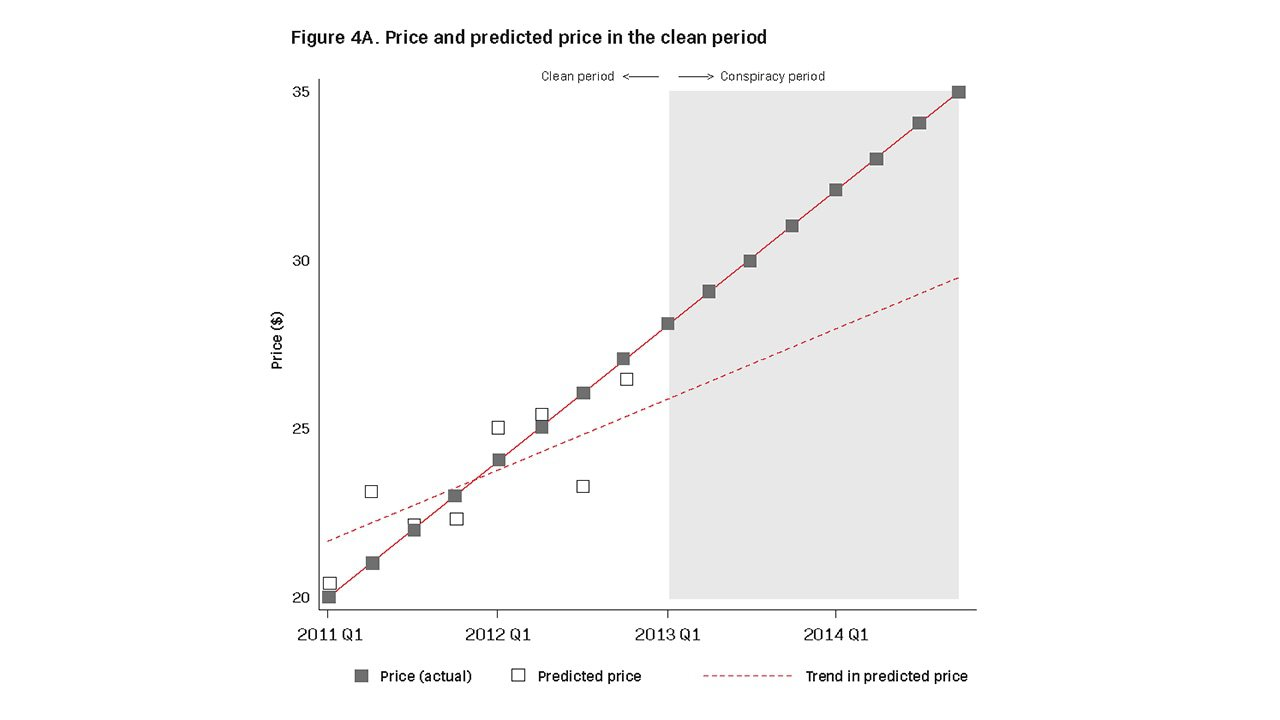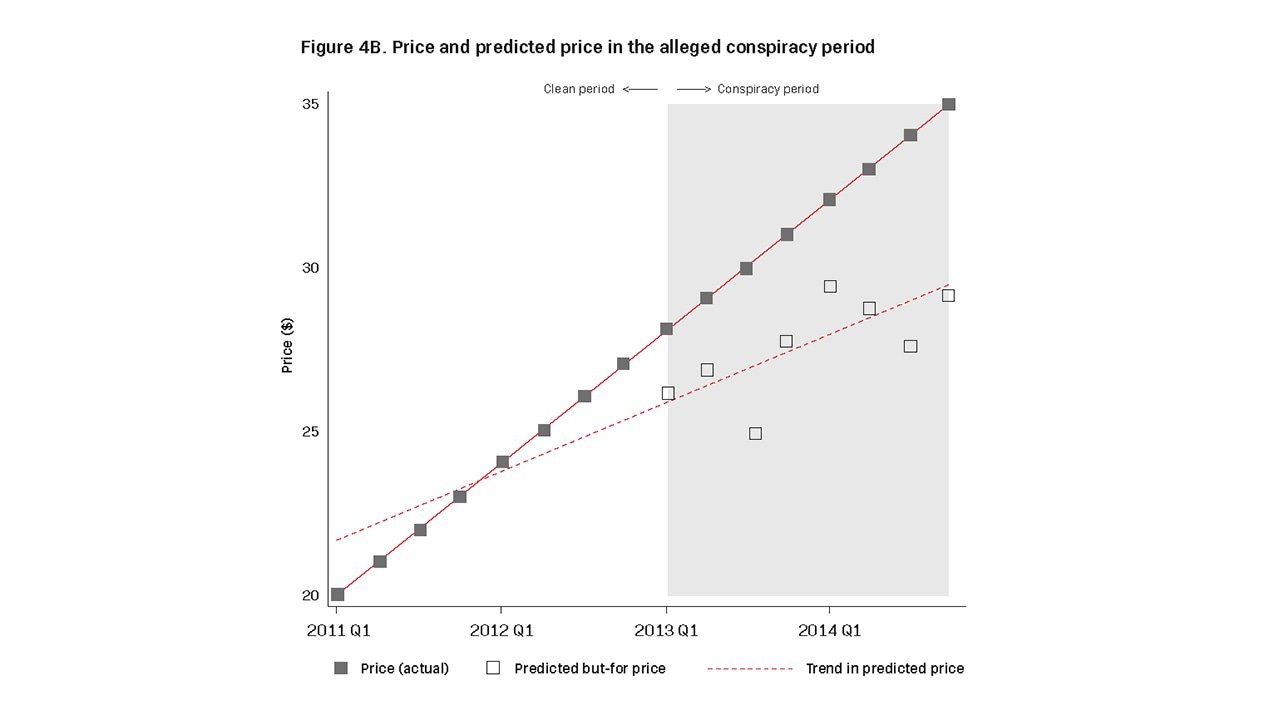In our stylised example, there is a positive correlation between cost and the conspiracy-period dummy because both are rising over time: cost rises over time by construction, and the conspiracy-period dummy rises from 0 in the clean period to 1 in the conspiracy period. A positive correlation between cost and the conspiracy-period dummy – and therefore a positive overcharge estimate – would also arise if costs were falling over time, but the alleged conspiracy occurred before the available ‘clean’ data (and therefore the conspiracy-period dummy fell from 1 to 0 over time). Conversely, in our stylised example, a negative correlation between cost and the conspiracy-period dummy – and therefore a negative bias on the overcharge estimate – would arise if costs were falling over time and the conspiracy period followed the clean period, or if costs were rising over time and the conspiracy period preceded the clean period. Table 2 summarises how trends in costs and the relative timing of the clean and conspiracy periods combine to produce a positive or negative bias on overcharge estimates in our simple, stylised scenario. Note that these stylised results ignore complications that may arise with a more complex model or data situation, and that such complications may enhance, cancel or drown out the effect of cost mismeasurement.

Table 2. Sign of bias in overcharge estimate in simple prediction or dummy-variable model

Trend in cost over timeTiming of clean period relative to conspiracy period
BeforeAfter
Increasing+-
Decreasing-+
Note: These results apply when costs are always increasing or always decreasing and the expert uses (1) a prediction model based on a regression of price on cost or (2) a simple dummy-variable model in which price is regressed on cost and a conspiracy-period dummy. The sign of the overcharge-estimate bias may vary if the behaviour of the data is less straightforward or the expert uses a more complex model.

## Discussion

Whether the expert opts for the prediction approach or the dummy-variable approach, the implication of measurement error on this stylised example is the same: if cost is higher during the alleged conspiracy period than during the clean period, then, all else equal, overcharge estimates will be biased upwards.11

This pattern may occur frequently in industries with trending costs. Many alleged and admitted price-fixing conspiracies, for example, have involved high-tech products whose cost of production falls over time.12 In such situations, if the clean data available to the expert comes from after the alleged conspiracy period, then both the prediction approach and the dummy-variable approach may overestimate the overcharge.

Abstracting from complications that may arise with a more complex model, the severity of the measurement error bias depends on the severity of the measurement error and the speed at which costs are changing. To illustrate this, Table 3 reports the estimated overcharge from variations of the stylised example above. In all these variations, the time span of the available data has been extended from four to 20 years, with the alleged conspiracy spanning the second half of the data. As the results show, the overcharge estimates tend to become more biased both as measurement error increases in average magnitude and as cost increases more quickly.

Table 3. Estimated overcharge as a percentage of actual price: Stylised example with variation in severity of measurement error and speed of cost increases

Prediction method
Mean magnitude of measurement error as a proportion of average true costQuarterly increase in true cost
\$0\$0.50\$1\$1.50\$2\$5\$10
00.0%0.0%0.0%0.0%0.0%0.0%0.0%
0.20.0%24.5%26.4%27.0%27.2%27.8%27.9%
0.40.0%34.6%40.8%43.3%44.6%47.3%48.2%
0.60.0%37.6%45.7%49.1%51.1%54.9%56.3%
0.80.0%38.8%47.7%51.6%53.8%58.3%60.0%
10.0%39.4%48.7%52.9%55.2%60.0%61.8%

Dummy-variable method
Mean magnitude of measurement error as a proportion of average true costQuarterly increase in true cost
\$0\$0.50\$1\$1.50\$2\$5\$10
00.0%0.0%0.0%0.0%0.0%0.0%0.0%
0.20.0%23.4%24.9%25.4%25.6%26.0%26.2%
0.40.0%34.0%39.8%42.1%43.3%45.8%46.6%
0.60.0%37.3%45.1%48.4%50.3%54.0%55.3%
0.80.0%38.6%47.3%51.2%53.3%57.7%59.3%
10.0%39.2%48.5%52.6%54.9%59.6%61.4%

We must stress, of course, that these examples reflect the simple scenario in which the only control variable is cost. In more complex models with additional control variables – which may or may not be measured with error themselves – the implications of measurement error may be more complicated. Measurement error may also have more complicated implications if the expert opts for a more complex estimation method.13 The expert may therefore need to think through the potential implications of measurement error on the particular situation and model. Even in more complex situations, however, the simple examples here may provide intuition about how measurement error in control variables may bias estimates of the overcharge.

## Approaches for addressing measurement-error bias

Naturally, the next question is how to prevent measurement-error bias in overcharge estimates, if indeed such bias can be prevented. The answer depends on the particular situation.

One potential solution to the measurement-error problem is an econometric technique called ‘instrumenting’ or the ‘instrumental variables method’. Instead of simply estimating the relationship between price and measured cost (for example), this method estimates the relationship between price and a part of measured cost that is explained by some other ‘instrumental’ variable. This part of measured cost should generally be uncorrelated with the measurement error, thereby eliminating or mitigating the problem of measurement-error bias. When it works, the instrumental variables method is an elegant solution.14 However, caveats apply. First, the method requires an appropriate instrumental variable, which may not be available.15 Second, if the instrumental variable used is not quite appropriate, instrumenting may be ineffective – or may even make the problem worse.16,17 Moreover, it may be difficult or impossible to verify whether instrumenting has been effective in addressing measurement-error bias.

Another potential solution to the measurement-error problem is to aggregate the data over time or other dimensions. For example, data available at the weekly level might be aggregated to the monthly or quarterly level; data corresponding to individual transactions might be aggregated to the product-family level. Aggregation may produce more accurate cost estimates if cost is, in fact, determined at a broader level (eg, over longer periods or larger product groups), with measurement error arising from the apportionment of costs to shorter periods or more specific product types. Aggregation may also produce more accurate cost estimates if the measurement error is random noise, as noise tends to cancel out as multiple observations are averaged together. For example, in a variation of our stylised example in which the data set is extended to cover 16 years rather than four, aggregating from quarterly to annual data reduces the dummy-variable method’s overcharge estimate from a statistically significant 11.0 per cent to a statistically insignificant 3.9 per cent.18

In some situations, therefore, aggregation may be an effective way to mitigate the problem of measurement error. It may also be a helpful tool in diagnosing measurement-error bias: large swings in overcharge estimates as the aggregation level varies may indicate that measurement-error bias is present, at least at the lower levels of aggregation. Moreover, if an overcharge estimate is initially sensitive to aggregation but stabilises as the level of aggregation continues to increase, then the estimate’s stability beyond some level of aggregation might signal that the measurement error problem has been effectively addressed. Note, however, that even when aggregation helps, it may not fully address measurement-error bias, and with some types of measurement error it will not mitigate the bias at all. Moreover, aggregation comes at a cost: the higher the level of aggregation, the smaller the number of data points available for estimating the overcharge model. In some situations, the aggregation necessary to address measurement-error bias may leave too few data points to reliably estimate the regression model.

Methods to alleviate measurement-error bias may also, in some cases, be suggested by industry knowledge or insights into the way prices are determined. In our stylised example, for instance, we know that price is set as a simple level (rather than proportional) markup over cost. Given this, we could avoid measurement-error bias by rearranging the overcharge regression to explain the price-cost margin (ie, price minus cost) rather than price itself. This price-cost margin, now the regression’s Y-variable, reflects the measurement error in cost, but it turns out that classical measurement error in a Y-variable does not lead to bias.19 In our stylised example, a regression of the price-cost margin on the conspiracy-period dummy yields a statistically insignificant overcharge estimate of only 0.2 per cent. Note, however, that the success of this approach depends on accurate knowledge of how prices and measurement error behave. If our knowledge were wrong, then the resulting misspecification of the model could lead to biased overcharge estimates.20

In sum, there is no one-size-fits-all solution to measurement-error bias. The appropriate response will depend on factors particular to the data, the overcharge regression model, and the industry setting. An expert witness should think through these factors thoroughly when attempting to estimate overcharges.

## Conclusion

As we have demonstrated, two common methods for estimating overcharges are vulnerable to bias when there is measurement error in a trending control variable. Given the prevalence of imperfectly measured control variables, experts should exercise vigilance when constructing their overcharge models, and consider whether measurement error is likely and what its effects would be on any particular model. Experts should also watch for warning signs of measurement-error problems, such as unexpectedly low-magnitude coefficients on cost (or other controls) or meaningful variation in overcharge estimates when the data is aggregated at different levels. In a model that allows for different overcharges in different sub-periods of the conspiracy period, another warning sign may be a ‘tell-tale staircase’ pattern: that is, a pattern of increasing or decreasing overcharge estimates that is unrelated to the pattern of alleged conduct.21

When measurement-error bias is suspected, there are a number of potential recourses. These include using the instrumental variables method, aggregating the data, and estimating a model that explains margins rather than prices. The appropriate choice will depend on the particular situation at hand – and may often be key to producing reliable overcharge estimates.

## Notes

1 Even if the expert has access to ‘production cost’ data produced by the firms, this data might not precisely reflect the relevant production costs for which the overcharge regression may need to control.

2 Mismeasurement of a variable in a regression may be called ‘measurement error’ or ‘errors in variables’. In this article we present a stylised example using what economists term ‘classical measurement error’, or measurement error that is unrelated both to the outcome of interest and to the true values of the explanatory variable or variables. In general, we use ‘measurement error’ in this article to refer to classical measurement error.

3 See, for example, Maurice D Levi, ‘Errors in the Variables Bias in the Presence of Correctly Measured Variables’, 41 Econometrica 985 (1973).

4 For the purpose of this example, we draw the measurement error from a uniform distribution. Relatively large measurement errors are used for illustrative purposes. Note, however, that even relatively small measurement errors can produce phantom overcharges.

5 See, eg, ABA Section of Antitrust Law, Proving Antitrust Damages: Legal and Economic Issues, 182 (3d ed. 2017).

6 Throughout this article, percentage overcharges are presented in terms of actual rather than but-for prices.

7 See, for example, Jeffrey M Woolridge, Introductory Econometrics: A Modern Approach, 320-22 (5th ed. 2013). Attenuation bias, also known as ‘regression dilution’, is caused by random, mean-zero measurement error in an X-variable of a regression. Note that attenuation bias is downwards in magnitude, pushing the estimated coefficient towards zero rather than negative infinity. In other words, if the true relationship between price and cost were negative, attenuation bias would push the estimated coefficient up towards zero.

8 The exception is explanatory variables that are uncorrelated both with the mismeasured variable and with all other explanatory variables that are correlated with the mismeasured variable. See, eg, Wooldridge (footnote 7, above), at 322.

9 This result also assumes a positive correlation between cost and price.

10 See, eg, Levi (footnote 3, above). Levi’s framework can be applied to derive the biases in a regression with two explanatory variables, one of which is mismeasured. In particular, the sign of the bias on the coefficient of the correctly measured explanatory variable is the product of (1) the sign of the correlation between the two explanatory variables and (2) the sign of the true coefficient on the mismeasured explanatory variable.

11 These implications apply to the simple prediction and dummy-variable models described above. In more complicated situations, with additional control variables or more sophisticated econometric techniques, the implications of measurement error may be more complicated.

12 Production costs, however, may not be the only costs relevant for pricing. In high-tech industries, for example, research and development costs tend to be large, and firms may account for these non-production costs in their pricing decisions. The appropriate treatment of non-production costs across a product’s life cycle may therefore be crucial to an accurate estimate of overcharge.

13 For example, the expert might opt for a ‘first difference’ approach, in which he or she seeks to explain changes in price using changes in cost. This approach (a ‘within’ approach) often exacerbates measurement-error bias. See, eg, Zvi Griliches and Jerry A Hausman, ‘Errors in Variables in Panel Data’, 31 Journal of Econometrics 93, 95 (1986).

14 In our stylised example, for instance, a second measure of cost could potentially serve as an instrument. When we construct such a measure (using the same procedure that generated the first cost measure) and implement the instrumental variables approach, the coefficient on cost rises from 0.56 to 0.71, and the estimated overcharge falls from a statistically significant \$3.54 to a statistically insignificant \$2.38.

15 We use ‘appropriate’ to mean not only that the instrument is, in technical parlance, relevant and exogenous, but also that it is sufficiently relevant (ie, not a weak instrument).

16 See, for example, Wooldridge (footnote 7, above), at 521 to 522.

17 For further discussion of the use of instrumental variables to address measurement-error bias, see, eg, Jerry Hausman, ‘Mismeasured Variables in Econometric Analysis: Problems from the Right and Problems from the Left’, 15 Journal of Economic Perspectives 57, 60, 61 (2001).

18 This extension of the data period means that after aggregation to the annual level, the observation count remains the same as in the original stylised example. The speed of cost increases is assumed to be lower, such that the overall cost increase in the 16-year data set is similar to that in the original four-year data set. As in the original stylised example, the alleged conspiracy period is assumed to begin halfway through the available data.

19 See, eg, Wooldridge (footnote 7, above), at 318 to 320.

20 For instance, if we mistakenly assumed a proportional rather than a level markup in our stylised example, we would estimate a statistically significant negative overcharge, -1.1 per cent (p = 0.043).

21 Such a pattern may arise because as the time to or from the clean period increases, the consequences of failing to adequately account for cost-driven price increases (or price increases driven by other mismeasured control variables) become more severe.